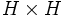# Conjugacy-closedness is not upper join-closed

This article gives the statement, and possibly proof, of a subgroup property (i.e., conjugacy-closed subgroup) not satisfying a subgroup metaproperty (i.e., upper join-closed subgroup property).
View all subgroup metaproperty dissatisfactions | View all subgroup metaproperty satisfactions|Get help on looking up metaproperty (dis)satisfactions for subgroup properties
Get more facts about conjugacy-closed subgroup|Get more facts about upper join-closed subgroup property|

## Statement

We can have the following situation:$H \le G$ is a subgroup,$K_1, K_2$ are intermediate subgroups of$G$ containing$H$, such that$H$ is conjugacy-closed in$K_1$ as well as in$K_2$, but not in the join$\langle K_1, K_2$.

## Proof

### A generic example

Suppose$H$ is a group with an automorphism$\sigma$ of order two that is not class-preserving: the automorphism does not preserve conjugacy classes. For instance,$H$ could be an Abelian group of exponent greater than two, and$\sigma$ could be the inverse map.

Let$A$ be the subgroup of$\operatorname{Aut}(H \times H)$ generated by the automorphism$(\sigma,\sigma)$ (i.e.,$\sigma$ acting coordinate-wise) and the coordinate exchange automorphism. Since both these automorphisms are of order two and commute,$A$ is a Klein-four group. Define$G = (H \times H) \rtimes A$ with the specified action.

Now, let$B_1$ be the two-element subgroup of$A$ generated by the coordinate exchange automorphism, and$B_2$ be the two-element subgroup of$A$ generated by the composite the coordinate exchange automorphism and$(\sigma,\sigma)$. Define$K_1 = (H \times H)B_1$ and$K_2 = (H \times H)B_2$.

• The subgroup$H = H \times \{ e \}$ is conjugacy-closed in$K_1$: Any element of$K_1$ is a product of an element in$H \times H$ and an element in$H \times H$. Note that the element of$H \times H$ preserves conjugacy classes, so it remains to see the effect of the element of$B_1$. if the element of$B_1$ is trivial, it of course acts as the identity. If it is not trivial, it sends every element of$H \times \{ e \}$ to an element of$\{ e \} \times H$, so no two distinct conjugacy classes in$H \times \{ e \}$ get fused by the action of$B_1$.
• The subgroup$H = H \times \{ e \}$ is conjugacy-closed in$K_1$: The reasoning is identical to the above reasoning.
• The subgroup$H = H \times \{ e \}$ is not conjugacy-closed in$G$: Indeed, the automorphism$(\sigma,\sigma)$ does not preserve conjugacy classes in$H \times \{ e \}$.

A similar kind of example can be constructed when the automorphism$\sigma$ is not class-preserving, and has finite order$n > 2$. In this case, we need to case a$n$-fold direct product of$H$, and have the diagonal automorphism$\sigma$ as well as the cyclic coordinate permutation automorphism act on this direct product.

### Some particular examples

The smallest particular example of the above is when$H$ is the cyclic group of order three, and$\sigma$ is the inverse map.$G$ in this case has order$36$, and$K_1, K_2$ both have order$18$.

Within nilpotent groups, the smallest particular example is when$H$ is the cyclic group of order four, and$\sigma$ is the inverse map.$G$ has order$64$, and$K_1, K_2$ both have order$32$.